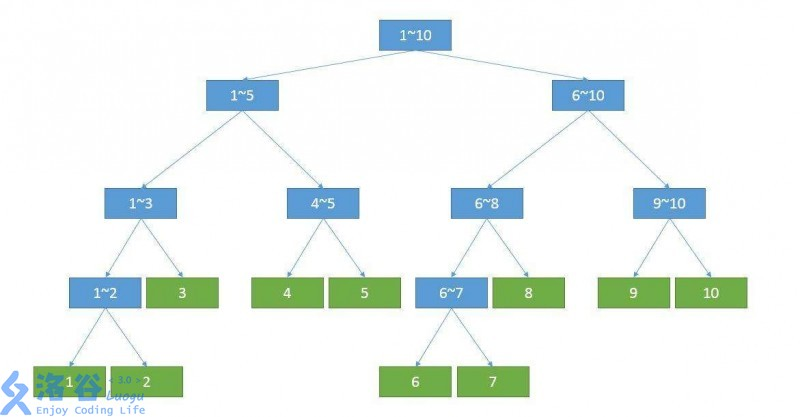# 简单数据结构——树状数组、线段树、哈希表

### 1.树状数组

​ 现阶段，树状数组主要用于维护序列前缀和　(其实还支持区间和、区间异或和、区间乘积和RMQ等具有交换律的问题)　。

#### 将$i$二进制分解，最小的二的次幂记为lowbit(i)

*树状数组求逆序对(二维偏序)洛谷P1908　　洛谷P1774

//定义数组，b[]为读入的数组，a[]为要离散化后的数组，c[]为树状数组
struct node
{int v,id;}a[N];
bool cmp(const node &x,const node &y)
{return x.v<y.v;}
int main()
{
scanf("%lld",&n);
for(int i=1;i<=n;i++)
scanf("%lld",&a[i].v),a[i].id=i;
sort(a+1,a+n+1,cmp);//按价值从大到小排序
int cnt=1;
for(int i=1;i<=n;i++)//离散化+去重
{
if(i!=1 &&a[i].v!=a[i-1].v)cnt++;
b[a[i].id]=cnt;
}
for(int i=1;i<=n;i++)
update(b[i],1),ans=ans+i-sum(b[i]);//因为是排完序之后，所以之前加入的一定比后加入的大
//然后在查询当前这个数前面位置的数，就是逆序对的个数了
printf("%lld\n",ans);
return 0;
}


​ 　*洛谷P3810(三位偏序，要用到CDQ分治) 还没弄懂

### 2.线段树

​ 线段树之所以称为“树”，是因为其具有树的结构特性。线段树由于本身是专门用来处理区间问题的（包括RMQ、RSQ问题等。)1.线段树每个节点都是一个区间

2.对于每一个区间为$[l,r]$非叶节点$k$，它的左儿子是$k<<1$,区间为$[l,mid]$，右儿子是$k<<1|1$，区间为$[mid+1,r]$。其中$mid=(l+r)>>1$

void pushdown(int k,int l,int r)//标记下传
{
int mid=(l+r)>>1;
}


*标记一个以上，记得考虑运算优先级！洛谷P3373

### 3.散列表(Hash)

​ 与离散化类似的是，Hash能把若干复杂的信息映射到一个容易维护的值域内，从而降低统计难度。哈希的过程，其实可以看作对一个串的单向加密过程，并且需要保证所加的密不能高概率重复

​ Hash算法应用较广泛，我只学习了 字符串哈希

​ 给定N个字符串（第i个字符串长度为Mi，字符串内包含数字、大小写字母，大小写敏感），请求出N个字符串中共有多少个不同的字符串。

long long hashe(char s[])
{
int len=strlen(s);
long long ans=0;
for (int i=0;i<len;i++)
ans=(ans*base+s[i])%mod+prime;   //核心操作，可类比十进制。
//＋prime可增加hash的可行度(不加本题可能会被卡哦)
return ans;						//mod 最好取一个较大的质数，作用同上。
}


1、无错哈希：挂链表之类的操作。

2、多重哈希：就是字面意思

Hash表与其说是一种数据结构，不如说是一种sao操作算法。它对于复杂信息的统计有着很大的应用。但是考试时其实Hash的应用不算特别多(我反正基本没用过)，而且哈希函数的选择也比较玄学，因此这里就不详细讲了。

posted @ 2018-11-24 09:49  Zerosking  阅读(187)  评论(0编辑  收藏